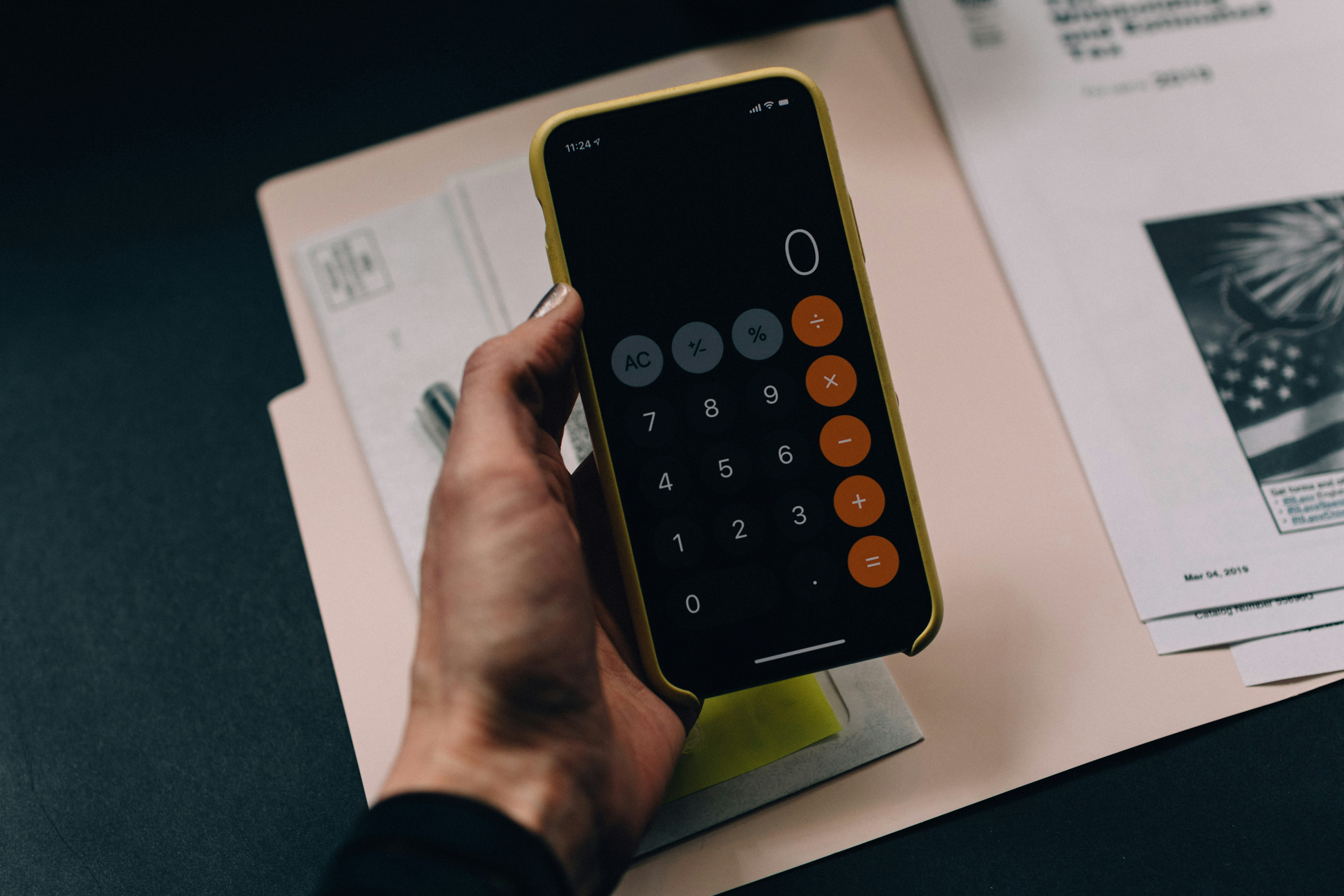Rambling Pete's Formula Reference Manual
Share
Explore#A reference manual for CODA functions

Note: The document is not complete yet. If you have a formula that you would like me to fast track, please let me know.
The idea behind this document is to provide extended working examples of how to use Coda formulas, both on the canvas and in tables.

- This is the main area of the document.
contains a searchable table with all the Coda formulas, as well as some Pack formulas.
contains a page for each formula, including some functioning examples on both the canvas, and in tables.
includes groups of formulas that are similar, for example all the rounding formulas. The page will then compare and contrast the formulas to allow you to find the exact formula for your needs.